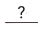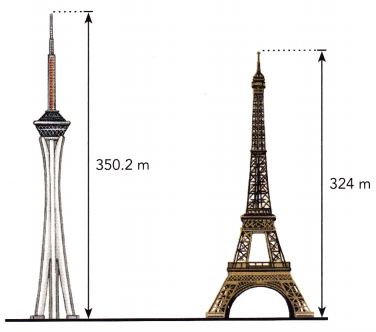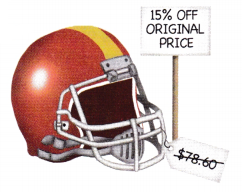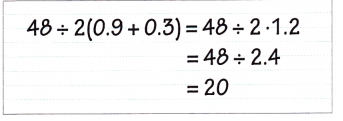# Math in Focus Grade 7 Chapter 2 Lesson 2.6 Answer Key Operations with Decimals

Go through the Math in Focus Grade 7 Workbook Answer Key Chapter 2 Lesson 2.6 Operations with Decimals to finish your assignments.

## Math in Focus Grade 7 Course 2 A Chapter 2 Lesson 2.6 Answer Key Operations with Decimals

### Math in Focus Grade 7 Chapter 2 Lesson 2.6 Guided Practice Answer Key

Copy and complete.

Question 1.
2.35 + (-6.13)
Using absolute values,=Subtract the lesser absolute value from the greater absolute value.
=Simplify.
2.35 + (-6.13) =Use asign, becausehas a greater absolute value.
2.35 – 6.13 = 6.13 – 2.35 Subtract the lesser absolute value from the greater absolute value.
= 3.78 Simplify.
2.35 + (-6.13) = 3.78 Use a negative sign, because 6.13 has a greater absolute value.

Solve.

Question 2.
-8.6 – 3.27
negative plus negative is negative
– × – = +
So we have to add the given numbers
-8.6 – 3.27 = -11.87

Question 3.
3.38 + (-5.6)
3.38 – 5.6 = 5.6 – 3.38
= 2.22
Th greatest number has negative sign so we have to put negative sign to the resultant numbr.
3.38 + (-5.6) = -2.22

Solve.

Question 4.
At noon, the temperature was 2.7°F. By midnight, the temperature had dropped 7.5°F. Then, during the morning, the temperature rose 3.8°F. Find the final temperature.
2.7 + (-7.5) + 3.8 = (2.7 + 3.8) + (-7.5)
= 6.5 + (-7.5), /6.5/</-7.5/, /-7.5/-/6.5/ = -1°F

Evaluate each product.

Question 5.
-7.23 • 4.6
-7.23 • 4.6
– × + = –
-7.23 • 4.6 = -33.258

Question 6.
-37 • (-9.2)
-37 • (-9.2)
– × – = +
-37 • (-9.2) = 340.4

Question 7.
8% of $230 Answer: Given, 8% of$230
8% = 8/100 = 0.08
0.08 × 230 = 18.4

Tell whether each quotient is positive or negative. Then evaluate the quotient.

Question 8.
-21.7 ÷ 0.7
As we know that – × + = –
The quotient will be negative.

Question 9.
-31.92 ÷ (-4.2)
We know that -×-=+. So the quotient will be positive.
First we have to cancel negative signs.
31.92 ÷ 4.2 = 7.6

Solve.

Question 10.
The temperature in a certain town drops by 1.6°F per hour for 1.2 hours. It then drops by 0.8°F per hour for 2.5 hours. Find the total change in temperature.
You can use a negative number to represent the hourly drop in temperature.Total change in temperature:
1.6 •+ (-0.8) •=+=The total change in temperature is°F.

Total change in temperature:
1.6 • 1.2 + (-0.8) • 2.5 = 1.92 + (-2)
= -0.8
The total change in temperature is -0.8 °F.

Question 11.
Mrs. Thompson usually uses 0.5 cup of sugar to bake a raisin cake. To bake a healthier raisin cake using less sugar, she decreases the amount of sugar by 20%. What ¡s the amount of sugar that Mrs. Thompson uses for baking the healthier raisin cake?
0.5 – () = 0.5 –Multiply.
=Add.
The amount of sugar that she uses iscup.
0.5 – (0.5 • 0.2) = 0.5 – 0.1 Multiply.
= 0.4

Question 12.
Janice buys four boxes of paper clips. She pays with a $10 bill and receives$6.08 in change. What is the price of one box of paper clips?
Given,
Janice buys four boxes of paper clips. She pays with a $10 bill and receives$6.08 in change.
10 – 6.08 = 3.92
3.92 ÷ 4 = 0.98
Thus the cost of each paper clip is $0.98 Question 13. According to the 2000 census and the 2010 census, the population of the United States changed from 281.4 million to 308.7 million. Find the percent change in the population of the United States over the 10-year period. Round your answer to the nearest tenth. Answer: Given, According to the 2000 census and the 2010 census, the population of the United States changed from 281.4 million to 308.7 million. 308.7 – 281.4 = 27.3 27.3/281.4 = 0.97 = 9.7% 9.7 to the nearest tenth is 10. Question 14. Stratosphere Tower in Las Vegas, Nevada, is the tallest freestanding observation tower in United States. Its height is 350.2 meters. The Eiffel Tower in Paris, France, is the country’s tallest building. Its height ¡s 324 meters. Find the difference in their heights.Answer: Given, Stratosphere Tower in Las Vegas, Nevada, is the tallest freestanding observation tower in United States. Its height is 350.2 meters. The Eiffel Tower in Paris, France, is the country’s tallest building. Its height is 324 meters. 350.2 – 324 = 26.2 meters Thus the difference between the two towers is 26.2 meters. ### Math in Focus Course 2A Practice 2.6 Answer Key Solve. Show your work. Evaluate each sum or difference. Question 1. -6.25 + 3.9 Answer: 6.25 – 3.9 = 2.35 Here the greatest value has negative sign so the difference of two numbers is -2.35 thus -6.25 + 3.9 = -2.35 Question 2. -2.074 + 1.8 Answer: 2.074 – 1.8 = 0.274 Here the greatest value has a negative sign so the difference of the two numbers is -0.274 Question 3. -11.52 – 6.3 Answer: The signs of both the numbers are same so we have to add the numbers. 11.52 + 6.3 = 17.82 -11.52 – 6.3 = -17.82 Question 4. -29.4 – (-7.21) Answer: -29.4 + 7.21 = 29.4 – 7.21 = 22.19 Here the greatest value has negative sign so the difference of two numbers is -22.19 Question 5. -8.106 – 0.98 Answer: The signs of both the numbers are same so we have to add the numbers. 8.106 + 0.98 = 9.086 Now put the negative sign to the answer. -8.106 – 0.98 = -9.086 Evaluate each product. Question 6. 0.3 • (-4.8) Answer: Plus × minus = minus 0.3 • (-4.8) = -1.44 Question 7. -1.6 • 2.9 Answer: Plus × minus = minus 1.6 × 2.9 = 4.64 Now put negative sign to the resultant. -1.6 • 2.9 = -4.64 Question 8. -3.25 • (-1.7) Answer: Minus × minus = plus 3.25 × 1.7 = 5.525 Question 9. 2.03 • (-5.4) Answer: Plus × minus = minus 2.03 × 5.4 = 10.962 Now put negative sign to the resultant. 2.03 • (-5.4) = -10.962 Question 10. -0.08 • 3.2 Answer: Plus × minus = minus 0.08 • 3.2 = 0.256 Now put negative sign to the resultant. -0.08 • 3.2 = -0.256 Evaluate each quotient. Question 11. -29.52 ÷ 3.6 Answer: 29.52 ÷ 3.6 = 8.2 Now put negative sign to the quotient -29.52 ÷ 3.6 = -8.2 Thus the quotient is -8.2 Question 12. 107.64 ÷ (-2.3) Answer: 107.64 ÷ (-2.3) Now put negative sign to the quotient 107.64 ÷ (2.3) = 46.8 107.64 ÷ (-2.3) = -46.8 Thus the quotient is -46.8 Question 13. -40.56 ÷ (-5.2) Answer: -40.56 ÷ (-5.2) = 40.56 ÷ 5.2 = 7.8 Thus the quotient is 7.8 Question 14. 9.758 ÷ 0.41 Answer: Given, 9.758 ÷ 0.41 = 23.8 Thus the quotient is 23.8 Evaluate each expression. Question 15. -0.59 – 1.2 – 3.4 Answer: All the signs in the given expression is same. So we have to add the numbers. 0.59 + 1.2 + 3.4 = 5.19 Now put the negative sign to the obtained answer. -0.59 – 1.2 – 3.4 = -5.19 Question 16. -2.38 + 15.6 – 140.05 Answer: Given, -2.38 + 15.6 – 140.05 -2.38 – 140.05 + 15.6 -142.43 + 15.6 =-126.83 Question 17. 38.92 – 6.7 – (-12.04) Answer: Given, 38.92 – 6.7 – (-12.04) 38.92 – 6.7 + 12.04 38.92 + 12.08 – 6.7 51 – 6.7 = 44.3 Question 18. 712.14 – 356.8 – (-9.03) Answer: Given, 712.14 – 356.8 – (-9.03) 712.14 – 356.8 + 9.03 First add the positive numbers 712.14 + 9.03 – 356.8 721.17 – 356.8 = 364.37 Question 19. 3 11.3 – 5.1 + 3.1 • 0.2 – 1.1 Answer: Given, 3 11.3 – 5.1 + 3.1 • 0.2 – 1.1 311.3 – 5.1 + 0.62 – 1.1 311.3 + 0.62 – 5.1 – 1.1 311.3 + 0.62 – 6.2 305.72 Question 20. (29.3 + 4) ÷ 3 + 0.5 • 2 Answer: Given, (29.3 + 4) ÷ 3 + 0.5 • 2 (33.3) ÷ 3 + 0.5 • 2 11.1 + 1 12.1 Solve. Show your work. Question 21. In Arizona, a minimum temperature of -40.0°F was recorded at Hawley Lake in 1971. A maximum recorded temperature of 53.3°F was recorded at Lake Havasu City in 1994. Find the difference between these maximum and minimum temperatures. Answer: Given, A minimum temperature of -40.0°F was recorded at Hawley Lake in 1971 A maximum recorded temperature of 53.3°F was recorded at Lake Havasu City in 1994. The difference between these maximum and minimum temperatures is 53.3°F – (-40.0°F) 53.3°F + 40°F 93.3°F Question 22. A shop owner buys 5 handbags to sell in his shop. The owner pays$39.75 for each handbag. Later, the owner has to sell the handbags at a loss. If he charges $27.79 for each handbag, what is his total loss for the 5 handbags? Answer: Given, A shop owner buys 5 handbags to sell in his shop. The owner pays$39.75 for each handbag.
5 x 39.75 = 198.75
Later, the owner has to sell the handbags at a loss.
he charges $27.79 for each handbag. 5 x 27.79 = 138.95 198.75 – 138.95 = 59.80 His total loss is$59.80

Question 23.
The original price of a football helmet was $78.60. If Peter was given a 15% discount, how much did he pay for the football helmet?Answer: Given, The original price of a football helmet was$78.60.
First, you have to find out what one percent of the price is by dividing 78.6 by 100.
One percent is 0.786.
You want to know what 15 percent of the price is so that you can subtract this as a discount, you do this by multiplying 0.786 by 15.
78.6 – 11.79 = 66.81
Thus peter pays $66.81 for the football helmet. Question 24. The state sales tax in New York is 4.25%. Jean spends$208 at a department store, but only half of the merchandise she purchases is taxed. What is her total bill?
Given,
The total amount of money spent by her = $208 Tax rate = 4.25% The amount that was taxed = half of the merchandise bought by her. Total bill = Initial bill + Tax amount Tax = (Half of money spend by her * 4.25)/100 Tax = (208/2 x 4.25)/100 Tax = 104 x 4.25/100 Tax = 1.04 x 4.25 =$4.42
Thus the total amount of bill = initial bill + tax amount = $208 +$ 4.42 = $212.42 Question 25. Sarah bought some T-shirts and a pair of shorts for$66.30. The pair of shorts costs $15.90 and each T-shirt costs$5.60. How many T-shirts did she buy?
Given,
Sarah bought some T-shirts and a pair of shorts for $66.30. The pair of shorts costs$15.90 and each T-shirt costs $5.60 66.3 – 15.9 = 50.4. 50.4 ÷ 5.6 = 9 Thus the Sarah bought 9 T-shirts Question 26. Margaret wants to buy 10 books. Six of them cost$12.50 each and the rest cost $26.35 each. If she only has$150, how much is she short?
Given,
Margaret wants to buy 10 books.
Six of them cost $12.50 each and the rest cost$26.35 each.
6 x 12.50=75
4 x 26.35=105.4
75 + 105.4=180.4
150 – 180.4= -30.4

Question 27.
The recommended calcium intake for men and women is about 1.2 grams per day. A glass of milk contains about 0.27 gram of calcium. If a man drinks 3 glasses of milk, how much additional calcium does he need from other food sources?
Given,
The recommended calcium intake for men and women is about 1.2 grams per day.
A glass of milk contains about 0.27 grams of calcium.
0.27 x 3 is 0.60 + 0.21=0.81
then 1.2-0.81=0.39

Question 28.
Math Journal Susannah evaluated an expression as follows:She made a common mistake when applying the order of operations. Explain her mistake and help her solve the problem correctly.
48 ÷ 2(0.9 + 0.3)
48 ÷ 2 (0.12)
48 ÷ 0.6 = 80

Question 29.
One day in February, the temperature at 9 A.M. is -6.8°F. At 3 P.M. on the same day, the temperature is 1.72°F.
a) Find the change in temperature.
One day in February, the temperature at 9 A.M. is -6.8°F. At 3 P.M.
On the same day, the temperature is 1.72°F.
-6.8 + 1.72 = -5.08
Thus the change in temperature is -5.08°F.

b) Find the average hourly rate of change in temperature.

question 30.
A submarine was cruising at 1,328.4 feet below sea level. It then rose at a rate of 14.76 feet per minute for 15 minutes.
a) Find the submarine’s depth after it rose for 15 minutes.
Let us first calculate the total number of feet the submarine rose in 15 minutes using the rate of rise.
rate = 14.76 feet per minute
This means that at every minute, the submarine rose by 14.76 feet.
1 minute = 14.76 feet
Therefore 15 minutes = 14.76 × 15 = 221.4 feet

b) If the submarine continued to rise at this same rate, find the time it took to reach the surface from the depth you found in a).
To find the new depth, we will subtract the number of feet risen from the initial depth.
initial depth = 1,328.4 feet
rise after 15 minutes = 221.4 feet
New depth = 1,328.4 – 221.4 = 1,107 feet

Question 31.
James is mountain climbing. Using a rope, James climbs down from the top of a steep cliff for 4 minutes at a rate of 12.2 feet per minute. He then climbs back up for 10 minutes at a rate of 3.6 feet per minute. How far from the top of the cliff is he after 14 minutes?
Given,
James is mountain climbing. Using a rope, James climbs down from the top of a steep cliff for 4 minutes at a rate of 12.2 feet per minute.
12.2 × 4 = 48.8
He then climbs back up for 10 minutes at a rate of 3.6 feet per minute.
3.6 × 10 = 36
48.8 + 36 = 84.8 feet

Question 32.
Salina has 120 shares in a shipyard company. On Monday, the value of each share dropped by $0.38. On Tuesday, the value of each share rose by$0.16. Find the total change in the value of Salina’s 120 shares.
Given,
Salina has 120 shares in a shipyard company.
On Monday, the value of each share dropped by $0.38. On Tuesday, the value of each share rose by$0.16.
120(0.38 + 0.16)
120 (0.54) = $64.8 Question 33. An organization that raises funds for charity raised$2.45 million last year and $1.96 million this year. Find the percent change in the amount raised. Answer: Given, An organization that raises funds for charity raised$2.45 million last year and $1.96 million this year. 2.45 – 1.96 =$ 0.49 million
Thus the percent change in the amount raised is $0.49 million Question 34. A company suffered a loss of$5.4 million in its first year. It lost another $3.1 million in the second year. It made a profit of$4.9 million in the third year.

a) Find the average profit or loss for the first three years.
A company suffered a loss of $5.4 million in its first year. It lost another$3.1 million in the second year.
$5.4 –$3.1 = $2.3 million It made a profit of$4.9 million in the third year.
$4.9 –$2.3 = $2.6 million b) After its fourth year of business, the company’s combined profit for all four years was$0. Find the company’s profit or loss during the fourth year.
Let the Company’s loss or profit for the fourth year be x
$Company\ combined\ profit\ for\ all\ the\ fourth years\ is\$0
-5.4 – 3.1 + 4.9 + x = 0
-36 + x = 0 Simplify
-3.6 + x + 3.6 = 0 + 3.6 Add 3.6 to both sides
x = 3.6
Therefore,\ Company’s\ made\ a\ profit\ of\ $3.6\ million\ during\ the\ fourth\ year$

Brain Work

Question 1.
The average of seven rational numbers is -5.16.
a) Find the sum of the rational numbers.

b) If six of the numbers each equal -5.48, find the seventh number.

Question 2.
The temperature of dry air decreases by about 0.98°F for every 100-meter increase in altitude.
a) The temperature above a town is 16°F on a dry day. Find the temperature of an aircraft 3.6 kilometers above the town.

b) The temperature outside an aircraft 1.8 kilometers above the town is -5.64°F. Find the temperature in the town.

Question 3.
Julie finds a way to use mental math to find the averages of these numbers:
15, 19, 18, 12, 20
she guesses that the mean is about 17, and uses mental math to find how far above or below this value each data item is:
-2, 2, 1, -5,3
she uses mental math to add these amounts:
-2 + 2 + 1 + (-5) + 3 = -1
she then divides -1 by 5 to get an average of -0.2. she says this means that the average of the numbers is 0.2 less than 17, the number she estimated. Check that Julie’s method gives the correct average. Then use it to find the average of these 4 numbers:
32, 35, 38, 36